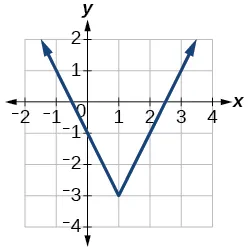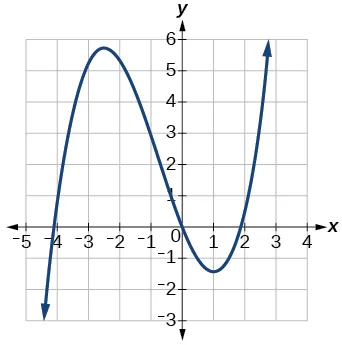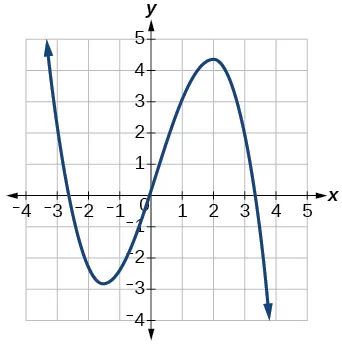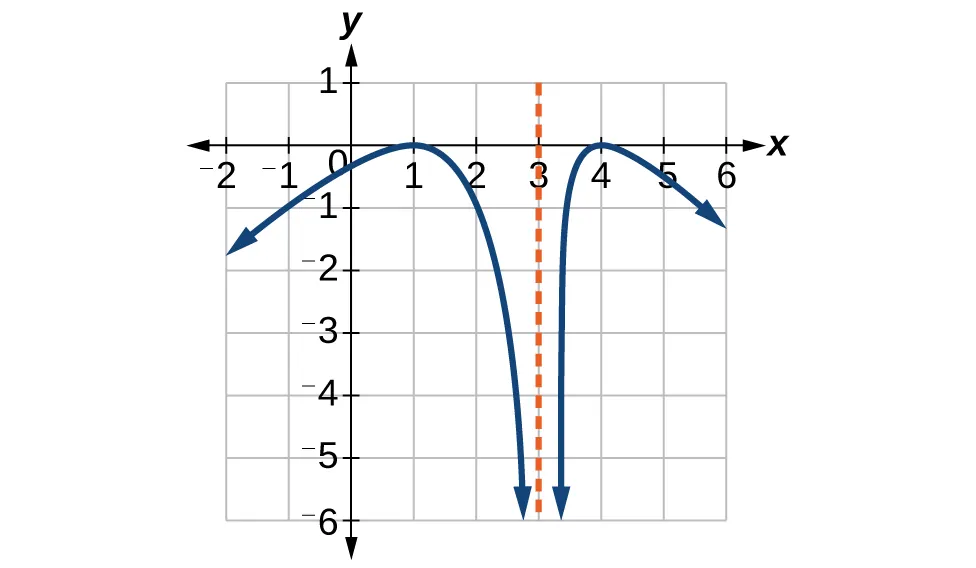College Algebra 2e

# 3.3Rates of Change and Behavior of Graphs

College Algebra 2e3.3 Rates of Change and Behavior of Graphs

### Learning Objectives

In this section, you will:

• Find the average rate of change of a function.
• Use a graph to determine where a function is increasing, decreasing, or constant.
• Use a graph to locate local maxima and local minima.
• Use a graph to locate the absolute maximum and absolute minimum.

Gasoline costs have experienced some wild fluctuations over the last several decades. Table 15 lists the average cost, in dollars, of a gallon of gasoline for the years 2005–2012. The cost of gasoline can be considered as a function of year.

 $y y$ 2005 2006 2007 2008 2009 2010 2011 2012 $C( y ) C( y )$ 2.31 2.62 2.84 3.3 2.41 2.84 3.58 3.68
Table 1

If we were interested only in how the gasoline prices changed between 2005 and 2012, we could compute that the cost per gallon had increased from $2.31 to$3.68, an increase of $1.37. While this is interesting, it might be more useful to look at how much the price changed per year. In this section, we will investigate changes such as these. ### Finding the Average Rate of Change of a Function The price change per year is a rate of change because it describes how an output quantity changes relative to the change in the input quantity. We can see that the price of gasoline in Table 1 did not change by the same amount each year, so the rate of change was not constant. If we use only the beginning and ending data, we would be finding the average rate of change over the specified period of time. To find the average rate of change, we divide the change in the output value by the change in the input value. The Greek letter $Δ Δ$ (delta) signifies the change in a quantity; we read the ratio as “delta-y over delta-x” or “the change in $y y$ divided by the change in $x. x.$” Occasionally we write $Δf Δf$ instead of $Δy, Δy,$ which still represents the change in the function’s output value resulting from a change to its input value. It does not mean we are changing the function into some other function. In our example, the gasoline price increased by$1.37 from 2005 to 2012. Over 7 years, the average rate of change was

On average, the price of gas increased by about 19.6¢ each year.

Other examples of rates of change include:

• A population of rats increasing by 40 rats per week
• A car traveling 68 miles per hour (distance traveled changes by 68 miles each hour as time passes)
• A car driving 27 miles per gallon (distance traveled changes by 27 miles for each gallon)
• The current through an electrical circuit increasing by 0.125 amperes for every volt of increased voltage
• The amount of money in a college account decreasing by \$4,000 per quarter

### Rate of Change

A rate of change describes how an output quantity changes relative to the change in the input quantity. The units on a rate of change are “output units per input units.”

The average rate of change between two input values is the total change of the function values (output values) divided by the change in the input values.

$Δy Δx = f( x 2 )−f( x 1 ) x 2 − x 1 Δy Δx = f( x 2 )−f( x 1 ) x 2 − x 1$

### How To

Given the value of a function at different points, calculate the average rate of change of a function for the interval between two values $x 1 x 1$ and $x 2 . x 2 .$

1. Calculate the difference $y 2 − y 1 =Δy. y 2 − y 1 =Δy.$
2. Calculate the difference $x 2 − x 1 =Δx. x 2 − x 1 =Δx.$
3. Find the ratio $Δy Δx . Δy Δx .$

### Example 1

#### Computing an Average Rate of Change

Using the data in Table 1, find the average rate of change of the price of gasoline between 2007 and 2009.

#### Analysis

Note that a decrease is expressed by a negative change or “negative increase.” A rate of change is negative when the output decreases as the input increases or when the output increases as the input decreases.

### Try It #1

Using the data in Table 1, find the average rate of change between 2005 and 2010.

### Example 2

#### Computing Average Rate of Change from a Graph

Given the function $g( t ) g( t )$ shown in Figure 1, find the average rate of change on the interval $[ −1,2 ]. [ −1,2 ].$

Figure 1

#### Analysis

Note that the order we choose is very important. If, for example, we use $y 2 − y 1 x 1 − x 2 , y 2 − y 1 x 1 − x 2 ,$ we will not get the correct answer. Decide which point will be 1 and which point will be 2, and keep the coordinates fixed as $( x 1 , y 1 ) ( x 1 , y 1 )$ and $( x 2 , y 2 ). ( x 2 , y 2 ).$

### Example 3

#### Computing Average Rate of Change from a Table

After picking up a friend who lives 10 miles away and leaving on a trip, Anna records her distance from home over time. The values are shown in Table 2. Find her average speed over the first 6 hours.

 t (hours) 0 1 2 3 4 5 6 7 D(t) (miles) 10 55 90 153 214 240 292 300
Table 2

#### Analysis

Because the speed is not constant, the average speed depends on the interval chosen. For the interval [2,3], the average speed is 63 miles per hour.

### Example 4

#### Computing Average Rate of Change for a Function Expressed as a Formula

Compute the average rate of change of $f( x )= x 2 − 1 x f( x )= x 2 − 1 x$ on the interval $[2,4]. [2,4].$

### Try It #2

Find the average rate of change of $f( x )=x−2 x f( x )=x−2 x$ on the interval $[1,9]. [1,9].$

### Example 5

#### Finding the Average Rate of Change of a Force

The electrostatic force $F, F,$ measured in newtons, between two charged particles can be related to the distance between the particles $d, d,$ in centimeters, by the formula $F( d )= 2 d 2 . F( d )= 2 d 2 .$ Find the average rate of change of force if the distance between the particles is increased from 2 cm to 6 cm.

### Example 6

#### Finding an Average Rate of Change as an Expression

Find the average rate of change of $g( t )= t 2 +3t+1 g( t )= t 2 +3t+1$ on the interval $[0,a]. [0,a].$ The answer will be an expression involving $a a$ in simplest form.

### Try It #3

Find the average rate of change of $f(x)= x 2 +2x−8 f(x)= x 2 +2x−8$ on the interval $[5,a] [5,a]$ in simplest forms in terms of $a. a.$

### Using a Graph to Determine Where a Function is Increasing, Decreasing, or Constant

As part of exploring how functions change, we can identify intervals over which the function is changing in specific ways. We say that a function is increasing on an interval if the function values increase as the input values increase within that interval. Similarly, a function is decreasing on an interval if the function values decrease as the input values increase over that interval. The average rate of change of an increasing function is positive, and the average rate of change of a decreasing function is negative. Figure 3 shows examples of increasing and decreasing intervals on a function.

Figure 3 The function $f( x )= x 3 −12x f( x )= x 3 −12x$ is increasing on $( −∞,−2 ) ∪ ​ ​ ( 2,∞ ) ( −∞,−2 ) ∪ ​ ​ ( 2,∞ )$ and is decreasing on $(−2,2). (−2,2).$

While some functions are increasing (or decreasing) over their entire domain, many others are not. A value of the input where a function changes from increasing to decreasing (as we go from left to right, that is, as the input variable increases) is the location of a local maximum. The function value at that point is the local maximum. If a function has more than one, we say it has local maxima. Similarly, a value of the input where a function changes from decreasing to increasing as the input variable increases is the location of a local minimum. The function value at that point is the local minimum. The plural form is “local minima.” Together, local maxima and minima are called local extrema, or local extreme values, of the function. (The singular form is “extremum.”) Often, the term local is replaced by the term relative. In this text, we will use the term local.

Clearly, a function is neither increasing nor decreasing on an interval where it is constant. A function is also neither increasing nor decreasing at extrema. Note that we have to speak of local extrema, because any given local extremum as defined here is not necessarily the highest maximum or lowest minimum in the function’s entire domain.

For the function whose graph is shown in Figure 4, the local maximum is 16, and it occurs at $x=−2. x=−2.$ The local minimum is $−16 −16$ and it occurs at $x=2. x=2.$

Figure 4

To locate the local maxima and minima from a graph, we need to observe the graph to determine where the graph attains its highest and lowest points, respectively, within an open interval. Like the summit of a roller coaster, the graph of a function is higher at a local maximum than at nearby points on both sides. The graph will also be lower at a local minimum than at neighboring points. Figure 5 illustrates these ideas for a local maximum.

Figure 5 Definition of a local maximum

These observations lead us to a formal definition of local extrema.

### Local Minima and Local Maxima

A function $f f$ is an increasing function on an open interval if $f( b )>f( a ) f( b )>f( a )$ for any two input values $a a$ and $b b$ in the given interval where $b>a. b>a.$

A function $f f$ is a decreasing function on an open interval if $f( b ) for any two input values $a a$ and $b b$ in the given interval where $b>a. b>a.$

A function $f f$ has a local maximum at $x=b x=b$ if there exists an interval $(a,c) (a,c)$ with $a such that, for any $x x$ in the interval $( a,c ), ( a,c ),$ $f( x )≤f( b ). f( x )≤f( b ).$ Likewise, $f f$ has a local minimum at $x=b x=b$ if there exists an interval $(a,c) (a,c)$ with $a such that, for any $x x$ in the interval $( a,c ), ( a,c ),$ $f( x )≥f( b ). f( x )≥f( b ).$

### Example 7

#### Finding Increasing and Decreasing Intervals on a Graph

Given the function $p( t ) p( t )$ in Figure 6, identify the intervals on which the function appears to be increasing.

Figure 6

#### Analysis

Notice in this example that we used open intervals (intervals that do not include the endpoints), because the function is neither increasing nor decreasing at $t=1 t=1$ , $t=3 t=3$ , and $t=4 t=4$ . These points are the local extrema (two minima and a maximum).

### Example 8

#### Finding Local Extrema from a Graph

Graph the function $f( x )= 2 x + x 3 . f( x )= 2 x + x 3 .$ Then use the graph to estimate the local extrema of the function and to determine the intervals on which the function is increasing.

#### Analysis

Most graphing calculators and graphing utilities can estimate the location of maxima and minima. Figure 8 provides screen images from two different technologies, showing the estimate for the local maximum and minimum.

Figure 8

Based on these estimates, the function is increasing on the interval $(−∞,−2.449) (−∞,−2.449)$ and $(2.449,∞). (2.449,∞).$ Notice that, while we expect the extrema to be symmetric, the two different technologies agree only up to four decimals due to the differing approximation algorithms used by each. (The exact location of the extrema is at $± 6 , ± 6 ,$ but determining this requires calculus.)

### Try It #4

Graph the function $f( x )= x 3 −6 x 2 −15x+20 f( x )= x 3 −6 x 2 −15x+20$ to estimate the local extrema of the function. Use these to determine the intervals on which the function is increasing and decreasing.

### Example 9

#### Finding Local Maxima and Minima from a Graph

For the function $f f$ whose graph is shown in Figure 9, find all local maxima and minima.

Figure 9

### Analyzing the Toolkit Functions for Increasing or Decreasing Intervals

We will now return to our toolkit functions and discuss their graphical behavior in Figure 10, Figure 11, and Figure 12.

Figure 10
Figure 11
Figure 12

### Use A Graph to Locate the Absolute Maximum and Absolute Minimum

There is a difference between locating the highest and lowest points on a graph in a region around an open interval (locally) and locating the highest and lowest points on the graph for the entire domain. The $y- y-$ coordinates (output) at the highest and lowest points are called the absolute maximum and absolute minimum, respectively.

To locate absolute maxima and minima from a graph, we need to observe the graph to determine where the graph attains it highest and lowest points on the domain of the function. See Figure 13.

Figure 13

Not every function has an absolute maximum or minimum value. The toolkit function $f( x )= x 3 f( x )= x 3$ is one such function.

### Absolute Maxima and Minima

The absolute maximum of $f f$ at $x=c x=c$ is $f( c ) f( c )$ where $f( c )≥f( x ) f( c )≥f( x )$ for all $x x$ in the domain of $f. f.$

The absolute minimum of $f f$ at $x=d x=d$ is $f( d ) f( d )$ where $f( d )≤f( x ) f( d )≤f( x )$ for all $x x$ in the domain of $f. f.$

### Example 10

#### Finding Absolute Maxima and Minima from a Graph

For the function $f f$ shown in Figure 14, find all absolute maxima and minima.

Figure 14

### Media

Access this online resource for additional instruction and practice with rates of change.

### 3.3 Section Exercises

#### Verbal

1.

Can the average rate of change of a function be constant?

2.

If a function $f f$ is increasing on $(a,b) (a,b)$ and decreasing on $(b,c), (b,c),$ then what can be said about the local extremum of $f f$ on $(a,c)? (a,c)?$

3.

How are the absolute maximum and minimum similar to and different from the local extrema?

4.

How does the graph of the absolute value function compare to the graph of the quadratic function, $y= x 2 , y= x 2 ,$ in terms of increasing and decreasing intervals?

#### Algebraic

For the following exercises, find the average rate of change of each function on the interval specified for real numbers $b b$ or $h h$ in simplest form.

5.

$f( x )=4 x 2 −7 f( x )=4 x 2 −7$ on $[1,b] [1,b]$

6.

$g( x )=2 x 2 −9 g( x )=2 x 2 −9$ on $[ 4,b ] [ 4,b ]$

7.

$p( x )=3x+4 p( x )=3x+4$ on $[2,2+h] [2,2+h]$

8.

$k( x )=4x−2 k( x )=4x−2$ on $[3,3+h] [3,3+h]$

9.

$f( x )=2 x 2 +1 f( x )=2 x 2 +1$ on $[x,x+h] [x,x+h]$

10.

$g( x )=3 x 2 −2 g( x )=3 x 2 −2$ on $[x,x+h] [x,x+h]$

11.

$a( t )= 1 t+4 a( t )= 1 t+4$ on $[9,9+h] [9,9+h]$

12.

$b( x )= 1 x+3 b( x )= 1 x+3$ on $[1,1+h] [1,1+h]$

13.

$j( x )=3 x 3 j( x )=3 x 3$ on $[1,1+h] [1,1+h]$

14.

$r( t )=4 t 3 r( t )=4 t 3$ on $[2,2+h] [2,2+h]$

15.

$f( x+h )−f( x ) h f( x+h )−f( x ) h$ given $f( x )=2 x 2 −3x f( x )=2 x 2 −3x$ on $[x,x+h] [x,x+h]$

#### Graphical

For the following exercises, consider the graph of $f f$ shown in Figure 15.

Figure 15
16.

Estimate the average rate of change from $x=1 x=1$ to $x=4. x=4.$

17.

Estimate the average rate of change from $x=2 x=2$ to $x=5. x=5.$

For the following exercises, use the graph of each function to estimate the intervals on which the function is increasing or decreasing.

18.19.20.21.For the following exercises, consider the graph shown in Figure 16.

Figure 16
22.

Estimate the intervals where the function is increasing or decreasing.

23.

Estimate the point(s) at which the graph of $f f$ has a local maximum or a local minimum.

For the following exercises, consider the graph in Figure 17.

Figure 17
24.

If the complete graph of the function is shown, estimate the intervals where the function is increasing or decreasing.

25.

If the complete graph of the function is shown, estimate the absolute maximum and absolute minimum.

#### Numeric

26.

Table 3 gives the annual sales (in millions of dollars) of a product from 1998 to 2006. What was the average rate of change of annual sales (a) between 2001 and 2002, and (b) between 2001 and 2004?

Year Sales
(millions of dollars)
1998201
1999219
2000233
2001243
2002249
2003251
2004249
2005243
2006233
Table 3
27.

Table 4 gives the population of a town (in thousands) from 2000 to 2008. What was the average rate of change of population (a) between 2002 and 2004, and (b) between 2002 and 2006?

Year Population
(thousands)
200087
200184
200283
200380
200477
200576
200678
200781
200885
Table 4

For the following exercises, find the average rate of change of each function on the interval specified.

28.

$f( x )= x 2 f( x )= x 2$ on $[1,5] [1,5]$

29.

$h( x )=5−2 x 2 h( x )=5−2 x 2$ on $[−2,4] [−2,4]$

30.

$q( x )= x 3 q( x )= x 3$ on $[−4,2] [−4,2]$

31.

$g( x )=3 x 3 −1 g( x )=3 x 3 −1$ on $[−3,3] [−3,3]$

32.

$y= 1 x y= 1 x$ on $[1,3] [1,3]$

33.

$p( t )= ( t 2 −4 )( t+1 ) t 2 +3 p( t )= ( t 2 −4 )( t+1 ) t 2 +3$ on $[−3,1] [−3,1]$

34.

$k( t )=6 t 2 + 4 t 3 k( t )=6 t 2 + 4 t 3$ on $[−1,3] [−1,3]$

#### Technology

For the following exercises, use a graphing utility to estimate the local extrema of each function and to estimate the intervals on which the function is increasing and decreasing.

35.

$f( x )= x 4 −4 x 3 +5 f( x )= x 4 −4 x 3 +5$

36.

$h( x )= x 5 +5 x 4 +10 x 3 +10 x 2 −1 h( x )= x 5 +5 x 4 +10 x 3 +10 x 2 −1$

37.

$g( t )=t t+3 g( t )=t t+3$

38.

$k( t )=3 t 2 3 −t k( t )=3 t 2 3 −t$

39.

$m( x )= x 4 +2 x 3 −12 x 2 −10x+4 m( x )= x 4 +2 x 3 −12 x 2 −10x+4$

40.

$n( x )= x 4 −8 x 3 +18 x 2 −6x+2 n( x )= x 4 −8 x 3 +18 x 2 −6x+2$

#### Extension

41.

The graph of the function $f f$ is shown in Figure 18.

Figure 18

Based on the calculator screen shot, the point $(1.333,5.185) (1.333,5.185)$ is which of the following?

1. a relative (local) maximum of the function
2. the vertex of the function
3. the absolute maximum of the function
4. a zero of the function
42.

Let $f(x)= 1 x . f(x)= 1 x .$ Find a number $c c$ such that the average rate of change of the function $f f$ on the interval $(1,c) (1,c)$ is $− 1 4 . − 1 4 .$

43.

Let $f( x )= 1 x f( x )= 1 x$ . Find the number $b b$ such that the average rate of change of $f f$ on the interval $(2,b) (2,b)$ is $− 1 10 . − 1 10 .$

#### Real-World Applications

44.

At the start of a trip, the odometer on a car read 21,395. At the end of the trip, 13.5 hours later, the odometer read 22,125. Assume the scale on the odometer is in miles. What is the average speed the car traveled during this trip?

45.

A driver of a car stopped at a gas station to fill up their gas tank. They looked at their watch, and the time read exactly 3:40 p.m. At this time, they started pumping gas into the tank. At exactly 3:44, the tank was full and the driver noticed that they had pumped 10.7 gallons. What is the average rate of flow of the gasoline into the gas tank?

46.

Near the surface of the moon, the distance that an object falls is a function of time. It is given by $d( t )=2.6667 t 2 , d( t )=2.6667 t 2 ,$ where $t t$ is in seconds and $d( t ) d( t )$ is in feet. If an object is dropped from a certain height, find the average velocity of the object from $t=1 t=1$ to $t=2. t=2.$

47.

The graph in Figure 19 illustrates the decay of a radioactive substance over $t t$ days.

Figure 19

Use the graph to estimate the average decay rate from $t=5 t=5$ to $t=15. t=15.$

### Footnotes

• 5http://www.eia.gov/totalenergy/data/annual/showtext.cfm?t=ptb0524. Accessed 3/5/2014.
Order a print copy

As an Amazon Associate we earn from qualifying purchases.